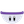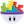#### Howdy, Stranger!

It looks like you're new here. If you want to get involved, click one of these buttons!

# Using TextReplace to change a formula and then calculate with it

Posts: 19Memberedited March 2016

Hi all,
I am working on a project that requires the user to enter a number, this number is then used to replace "X"s in formula pulled from a table using other info gleened from the users inputs for the table, row and column attributes.

I have successfully got this far using the TextReplace function and replacing any "X"s in the formulas in the table with the numbers entered by the user, and can then "Display" the new formula complete with the users number where the "X"s were.

Now I have that part tested and working I am struggling to find a way to actually calculate and display the result of that formula now it has the "X"s replaced by the users number, rather than displaying just the formula itself!

I know I'll probably kick myself for missing something obvious, but I really am stumped after many hours of trying all sorts to get this "simple" next and final step working, and once I figure this bit out I am virtually ready to publish, so I decided it's time to admit defeat and seek advice from the pro's!

So can anyone help before I go mad, bald or just give up!??

Thanks,
Andy P.

• Posts: 4,780Member, PROYou cannot use operations like / and * in a text string. If you want to evaluate the formulas, you'll need to break the formula apart more. So if you had:

(11-32)/1.8

you'd need to create an expression that looked like this:

(number1-number2)/number3

If you're using a string, it would end up

(textSubStr(string,2,3)-textSubStr(string,5,6))/textSubStr(string,9,11)

What you're doing is going to be very difficult in GameSalad and could potentially get hugely complicated.

• Posts: 11,845Member, Sous Chef, PRO, Senior Sous-ChefThe problem here is that you are entering mathematical formulas into your table cells. Table cells that contain text are just that: text. They are NOT expressions. They cannot use any of the string functions or math functions that you would use in the expression editor. You'll need to do all of your calculating and combining in a behavior's expression editor... not in the table cell directly.

It's tempting to think of a table as a spreadsheet because it looks like one but it's not a spreadsheet. It's really just a simple database (e.g. an array of records) without any calculation fields.

New to GameSalad? (FAQs)   |   Tutorials   |   Templates   |   Greenleaf Games   |   Educator & Certified GameSalad User

• Posts: 4,780Member, PROIs the formula fixed, with the units being used pulled from the table, or is the formula itself being pulled from the table?

• Posts: 11,845Member, Sous Chef, PRO, Senior Sous-Chefedited March 2016

If you store the result of the TextReplace function in a text attribute (using Change Attribute), you can then display or reuse that value as needed.

I also wonder if you're trying to enter values in the text portion of the Display Text field -- which will just show plain text such as "textReplace(...)" -- rather than clicking the 'e' to open the expression editor and then enter the functions and/or attributes there.

New to GameSalad? (FAQs)   |   Tutorials   |   Templates   |   Greenleaf Games   |   Educator & Certified GameSalad User

• Posts: 19Memberedited March 2016

Hi Guys and thank you for the rapid response, I really appreciate it.
@Armelline
The entire formula is being pulled from the table and then modified by replacing any "x"s in it with a number input into the Attribute InNum by the user instead.

@tatiang
I have tried storing the result in a text attribute as you suggest but am confused by the result not being as expected (see below) and yes I am using the expression editor as the resulting formula being displayed correctly when displaying the result of the equation directly will show.

Here is a walkthrough of what I am doing and the results I am getting, I hope it is clear enough;

Game Attributes;
Formula - text (to hold the formula from the table)
Formula2 - text (to hold the modified fomula with the users input number in place of the "x") so that it can be calculated to give the result of the formula with regard to the users input number (InNum)
InNum - real (The Attribute holding the users input number)

I have a Table called TemperatureTBL full of formulas such as; "(x-32)/1.8" where the "x" will be replaced by the number the user inputs into InNum

I have two Change Attribute behaviors;
1) set: game.Formula to: tableCellValue( game.TemperatureTBL, 2, 1+1)
2) set: game.Formula2 to: textReplace( game.Formula, "x", game.InNum )

immediately followed by a Display Text behavior.

If I set the Display Text behavior up like this;
display: textReplace( game.Formula, "x", game.InNum ) (ie the equation in #2 above directly placed in the display behavior)
it will display the formula from the table with the "x" replaced by the users input number (ie InNum) as intended (for test purposes).
(ie the display behavior displays "(11-32)/1.8" where "11" is the number that the user entered and is replacing the "x" that was in the original formula pulled from the table - as intended.)

But!

If I set the Display Text behavior to display game.Formula2 (which should have been set to the new formula with the "x" replaced by the users input number (ie InNum) because of the second Change Attribute above), the formula is displayed with the "x" replaced, but with 0 instead of the number input into InNum
(ie the display behavior displays "(0-32)/1.8" where "11" was the number that the user entered and should be replacing the "x" that was in the original formula pulled from the table - NOT as intended.)

I am baffled as to why displaying the result from the second Change Attribute behavior above is displaying different results to displaying the result of the equation directly rather than via the Attribute Formula2, which I assumed would be identical as it is supposed to be being set to the same result by using the same equation.

Until I figure out why the above results are not as expected I can not use game.Formula2 to calculate the answer/result of the user modified formula.

UPDATE:
I also just found that combining the two change attribute behaviors into one equation
( textReplace(tableCellValue( game.TemperatureTBL, 2, 1+1), "x", game.InNum ) )
turning the two change attribute behaviors off and putting that equation directly into the display behavior works as intended by displaying the modified formula correctly (ie it displays "(11-32)/1.8" where "11" was input by the user and replaced the "x" in the original formula pulled from the table), now if I could just work out how to display the calculated result of that formula instead of the actual formula I will have a fully functional App!

Am I missing something obvious or going about it in completely the wrong way?
It seemed such a simple thing to do when I started >.<

I hope you can help (and sorry for the "wall of text").

Thanks,
Andy P.

• Posts: 19MemberAnd thank you tatiang.